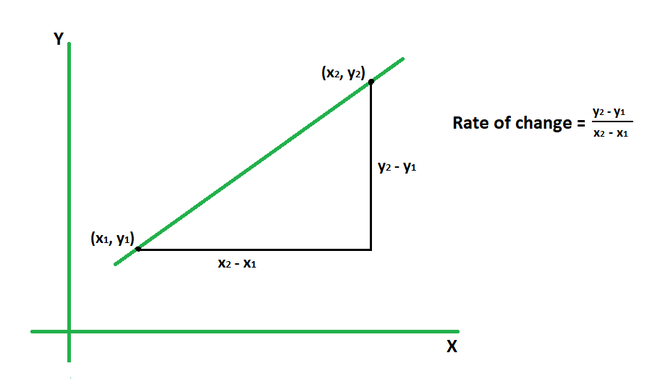# Rate of Change Formula

• Last Updated : 15 May, 2022

The rate of change of a variable quantity is defined as the rate at which it changes in relation to another quantity. To put it another way, the rate of change divides the amount of change in one variable by the equivalent amount of change in another variable. It describes the concept of how one quantity changes in proportion to the other. It has formulas defined for coordinates and linear functions as well.### Rate of Change Formula

The rate of change of coordinates (x1, y1) with respect to the other coordinates (x2, y2) is given by the ratio of the difference between the y-coordinates to that of the x-coordinates. In other words, the rate of change is equal to the slope of the line joining these points. It can also be denoted by the symbol m.

R = Δy/Δx = (y2 – y1) / (x2 – x1)

Where,

R is the rate of change of variable y with respect to variable x,

(x1, y1) and (x2, y2) are the coordinates.

For a linear function y = f(x), the rate of change is calculated for the interval (a, b) where b > a. Its formula is given by the ratio of the difference between the function values for each interval to the difference between interval values.

R = (f(b) – f (a)) / (b – a)

Here, R is the rate of change of variable y with respect to variable x for the interval (a, b).

### Sample problems

Problem 1: Calculate the rate of change for the coordinates (4, 11) and (2, 5).

Solution:

We have,

(x1, y1) = (4, 11)

(x2, y2) = (2, 5)

Using the formula, we have

R = (y2 – y1)/(x2 – x1)

= (5 – 11)/(2 – 4)

= -6/(-2)

= 3

Problem 2: The rate of change for two points (x, 3) and (1, 6) is 7. Find the value of x.

Solution:

(x1, y1) = (x, 3)

(x2, y2) = (1, 6)

R = 7

Using the formula, we have

R = (y2 – y1)/(x2 – x1)

=> 7 = (6 – 3)/(1 – x)

=> 7 = 3/(1 – x)

=> 7 – 7x = 3

=> 7x = 4

=> x = 4/7

Problem 3: The rate of change for two points (5, 4) and (3, y) is 4. Find the value of y.

Solution:

(x1, y1) = (5, 4)

(x2, y2) = (3, y)

R = 4

Using the formula, we have

R = (y2 – y1)/(x2 – x1)

=> 4 = (y – 4)/(3 – 5)

=> 4 = (y – 4)/(-2)

=> -8 = y – 4

=> y = -4

Problem 4: Calculate the rate of change for the function f(x) = x2 if the interval is (3, 5).

Solution:

We have, f(x) = x2

Calculate the value of f(3) and f(5).

f(3) = 32 = 9

f(5) = 52 = 25

Using the formula, we have

R = (f(b) – f(a))/(b – a)

= (f(5) – f(3))/ (5 – 3)

= (25 – 9)/2

= 16/2

= 8

Problem 5: Calculate the rate of change for the function f(x) = 4 – 3x3 if the interval is (1, 2).

Solution:

We have, f(x) = 4 – 3x3

Calculate the value of f(1) and f(2).

f(1) = 4 – 3(1)3 = 4 – 3 = 1

f(2) = 4 – 3(2)3 = 4 – 24 = -20

Using the formula,

R = (f(b) – f(a))/(b – a)

= (f(2) – f(1))/ (2 – 1)

= -20 – 1

= -21

Problem 6: The rate of change for two points (x, 7) and (9, 2) is 5. Find the value of x.

Solution:

(x1, y1) = (x, 7)

(x2, y2) = (9, 2)

R = 5

Using the formula,

R = (y2 – y1)/(x2 – x1)

=> 5 = (2 – 7)/(9 – x)

=> 5 = -5/(9 – x)

=> 45 – 5x = -5

=> 5x = 50

=> x = 10

Problem 7: The rate of change for two points (1, 5) and (8, y) is 9. Find the value of y.

Solution:

(x1, y1) = (1, 5)

(x2, y2) = (8, y)

R = 9

Using the formula,

R = (y2 – y1)/(x2 – x1)

=> 9 = (y – 5)/(8 – 1)

=> 9 = (y – 5)/7

=> y – 5 = 63

=> y = 68

My Personal Notes arrow_drop_up